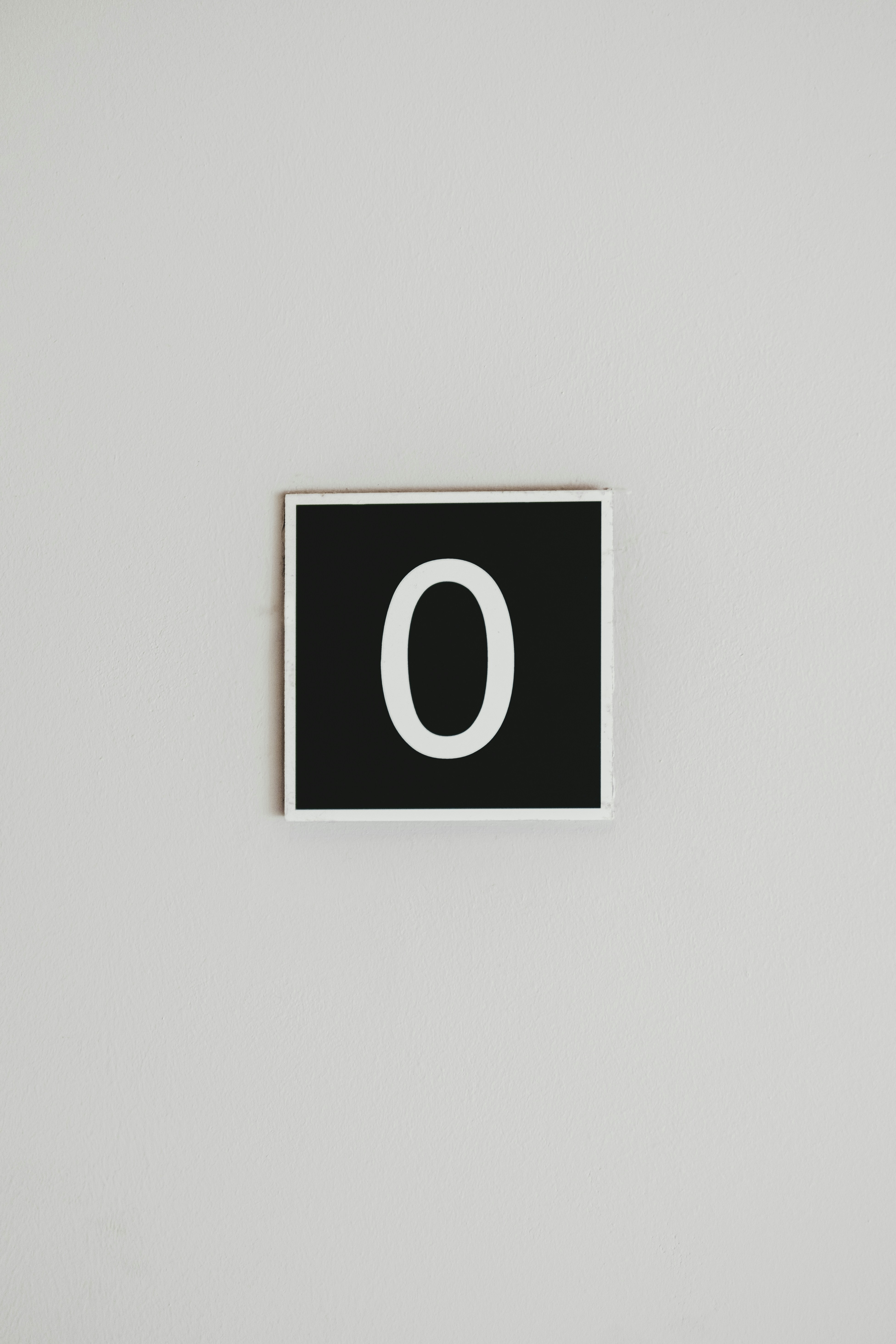Arsalan WasimConvolutional layers have the power to detect and learn various features in input images such as shapes, textures, and horizontal and vertical lines. One major problem with convolutional layers is that they miss the borders of the images. We can lose important information especially in smaller images where the size of the pictures is already small. To eliminate this problem, convolutiona; layers with padding are generally used.

As the name suggests, padding adds a border of zeros around the input image such that the border information is retained. Suppose that we have a two-dimensional matrix of size (three rows, three columns) such as:With padding this would look like:As you can see, our input image is now expanded with a pad of zeros such that it has become of size (five rows, five columns). You can also see that now our border information will also be preserved during the convolution operation because we have added an extra layer of zeros.

Now we will apply a convolution operation using a vertical line detector kernel like the following:Applying the convolution operation with our padded two-dimensional matrix we get:

0x0 + 1x0 + 0x0 + 0x0 +1x0 + 0x1 + 0x0 + 0x1 + 1x0 = 0

Now, moving the (3, 3) kernel to the right with a stride of one we get:

0x0 + 1x0 +0x0 + 0x0 +1x1 + 0x0 + 0x0 +1x1 + 0x0 = 2

Stride defines the amount of movement we need to move our kernel, in this case, it is one.

Again moving the kernel to the right, we will get 0.

We then move the kernel to the bottom such that the filter looks at second row.

Finally, we move the kernel to the right with a stride of one, giving us:

0x0 + 1x1 + 0x0 + 0x0 + 1x1 + 0x0 + 0x0 + 1x1 + 0x0 = 3

Similarly, we can do this for all the other numbers in the padded matrix until we get our output matrix, which is:We can see that the border information is maintained.